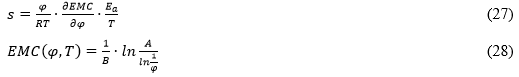Suchomelová, P., Trcala, M., and Tippner, J. (2019). "Numerical simulations of coupled moisture and heat transfer in wood during kiln drying: Influence of material nonlinearity," BioRes. 14(4), 9786-9805.

#### Abstract

Finite element simulations of coupled thermal and moisture fields in wood during kiln drying were observed with a focus on non-isothermal moisture transfer in three dimensional orthotropic models of wood with an initial moisture content below the fiber saturation point. Four different unsteady-state numerical models of the drying process were compared with the assumptions given by standards commonly used in wood kiln-drying processes. The first model describes linear simulation, and the other three models present nonlinear simulation using variable material coefficients dependent on temperature and moisture content, differing in settings of the Soret effect (thermodiffusion). A linear model was useful for predicting only the average moisture content during drying. Moreover, the nonlinear simulations were useful for computing the moisture content distribution. High differences (2.31% of moisture content) were found between the flow of moisture predicted by numerical models and standard requirements.

Numerical Simulations of Coupled Moisture and Heat Transfer in Wood during Kiln Drying: Influence of Material Nonlinearity

Pavlína Suchomelová,* Miroslav Trcala, and Jan Tippner

Finite element simulations of coupled thermal and moisture fields in wood during kiln drying were observed with a focus on non-isothermal moisture transfer in three‑dimensional orthotropic models of wood with an initial moisture content below the fiber saturation point. Four different unsteady-state numerical models of the drying process were compared with the assumptions given by standards commonly used in wood kiln-drying processes. The first model describes linear simulation, and the other three models present nonlinear simulation using variable material coefficients dependent on temperature and moisture content, differing in settings of the Soret effect (thermodiffusion). A linear model was useful for predicting only the average moisture content during drying. Moreover, the nonlinear simulations were useful for computing the moisture content distribution. High differences (2.31% of moisture content) were found between the flow of moisture predicted by numerical models and standard requirements.

Keywords: Coupled heat and mass transfer; Material coefficients nonlinearity; Multiphysical modeling; Kiln-drying wood

Contact information: Department of Wood Science, Faculty of Forestry and Wood Technology, Mendel University in Brno, Zemědělská 3, 613 00, Brno, Czech Republic;

* Corresponding author: pavlina.suchomelova@mendelu.cz

INTRODUCTION

Wood drying operations are an important part of lumber processing for use in the furniture or building industries. The quality of the drying process affects the final product quality, especially in terms of its physical and mechanical properties such as the dimensional stability, biodegradation resistance, or wood cracking. However, wood as a material shows significant property variability, and this adversely influences the drying process and dry timber quality (Pang 2007). The aim is to decrease drying time (and energy consumption) and the negative influences of drying on the properties of wood (Trcala 2015). One of the strategies on how to improve the process of wood drying is optimization of the drying schedule for different types of wood and for different requirements on the final product (Elustondo and Oliveira 2006). The drying and dry timber quality is affected by a wide range of factors, and thus it is costly and time consuming to experimentally investigate the influence of all these variables. Mathematical models have been recognized as a powerful tool to fulfil tasks of wood drying processes optimization (Pang 2004), and they can be also very useful for creating of drying schedules (Mitchell 2019) as well as for evaluation of stresses and cracks that arise during wood drying (Nascimento et al. 2019; Pérez-Pena et al. 2018) or for predicting of heat-mass transfer in wood during wood thermal modifications (He et al. 2019).

Wood drying is a dynamic process. It can be generally described as a coupled motion of the temperature and moisture fields with the local thermodynamic equilibrium in every point of the timber, reflecting the local changes of material coefficients depending on the mass and energy motion in the body (Gebhart 1993).

Kiln Drying of Wood

In terms of time and energy consumption, timber drying is one of the most demanding operations in wood processing (Elustondo and Oliveira 2006). The decreasing drying rate is compensated by continuous changes in the ambient temperature of drying (Jankowsky and Gonçalves Luiz 2006). Timber dries nonuniformly in its volume (Trcala 2012).

Convective kiln drying is the most commonly used method for sawn timber drying. The convectional kilns are designed to dry wood in the air and steam fusion, at the temperature of 40 °C (low-temperature seasoning and pre-drying) or temperatures of 40 to 100 °C (common hot-air drying) (Klement and Detvaj 2007; Perré and Keey 2007). As a good alternative to convective drying, superheated steam is also used as a drying medium (Kocaefe et al. 2007). Dehumidifier, vacuum, microwave, or solar kilns are other examples of wood drying (Perré and Keey 2007).

Modeling the Transport Processes in Wood

The numerical simulations of transport processes in wood are used as a tool for the mathematical and physical description of wood behavior in many aspects, such as building physics, behavior of wood-based composites, wood jointing, heat treatment of wood, or wood drying processes (Kang et al. 2008). The heat and mass modeling has progressed from the macroscopic description of these fields (Babiak 1995). The macroscopic model is based on the Fick’s law of diffusion for the mass transfer and the Fourier’s law for the heat transfer (Siau 1995). There are moisture concentration and temperature gradients in diffusion modeling, which are considered a moving force of the heat and mass transfer (Sherwood 1929; Vergnaud 1991). As a motion, the force of water diffusion in wood is sometimes used also as the water vapor partial pressure gradient (Siau 1984), where the air relative humidity (RH) influenced by actual moisture content in wood and temperature is observed. The multiphysical approach to the modeling of transport processes in wood seems to be the most appropriate to apply the thermodynamic models (Trcala 2012). The theory of coupled moisture and temperature fields motion is based on irreversible thermodynamic processes that were described by Luikov (1966) and Whitaker (1977).

Describing the bound moisture and temperature transfer in wood requires a comprehensive approach and the consideration of many physical phenomena. It is necessary to consider reciprocal influences of all the material coefficients and physical phenomena in coupled-fields modeling. Material properties of wood, such as density, diffusion coefficients, thermal conductivity coefficients, and specific heat, are dependent on the actual moisture content and temperature of wood (Horáček 2004). The weakness of most of the numerical models is their need for back-calculated material parameters without a clear physical meaning (Eitelberger and Hofstetter 2011).

The differences between full and partial nonlinearity of the heat and mass transfer in capillary-porous body were investigated by Lewis and Ferguson (1993). They compared two formulations of the Luikov equations (Luikov 1980). First, a fully nonlinear formulation with varying material properties, and second, a partially nonlinear formulation where some material properties were held constant. In the one‑dimensional example presented by Lewis and Ferguson (1993), the fully coupled model predicted the equilibrium achievement faster than the partial nonlinear model, but the difference was insignificant (Lewis and Ferguson 1993). This paper mainly presents a comparison of full nonlinear, partial nonlinear, and linear models of heat and mass transfer, regarding the Soret effect.

The heat and moisture transfer should be considered as coupled processes. The Soret effect (described by the thermodiffusion coefficient) is a moisture transfer induced by temperature gradient, and the Duffour effect expresses the heat flux induced by water diffusion (Siau 1984; Avramidis et al. 1992a).

It is not possible to use Fick’s and Fourier’s law separately, when modeling the wood drying processes. The multiphysical problem of water diffusion and heat transfer can be described by the system of two partial differential equations (PDEs), which is modified with relations to Soret and Duffour effects (Horáček 2004). When simulating the temperature and moisture fields during drying, the anisotropic or orthotropic properties of wood have to be considered (Trcala 2012).

Numerical models in this paper are focused on a convective hot-air kiln-drying of pre-dried timber. The study provides a comparison of numerical models using various settings of material parameters (different nonlinearity levels) and thermal-moisture fields couplings. Numerical solutions demonstrate that the settings of numerical model parameters and the coupling method can significantly influence simulation results, especially in its heating phase.

EXPERIMENTAL

Methods

The aim of the study was to determine the influence of nonlinearity of the material coefficients on the results of wood kiln-drying numerical models and to compare the results with commonly used standards for kiln‑drying of wood (ON 49 0651 1989) used in central Europe.

The software COMSOL Multiphysics (COMSOL AB, version 5.4, Stockholm, Sweden), which is based on the finite element method (FEM), was used for numerical solution. This software provides an opportunity to imply own PDEs systems and own material models.

Four various transient numerical models were built and are described in Table 1. In the first model (A) all of the material coefficients were set as constants. Material coefficients that depend on actual moisture content and temperature were used in simulations B1B2, and B3.

Table 1. Description of Four Variants of Numerical Models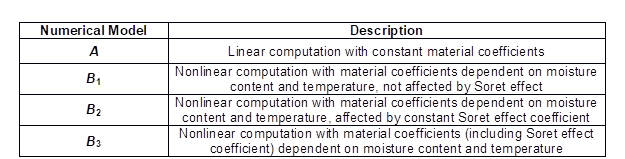For numerical modeling of wood drying processes it is necessary to define initial conditions, ambient parameters, boundary conditions, drying time, material coefficients of wood, and the region geometry. At the same time, it is important to consider the necessity of the simplification. These choices are important not only with respect to accurately modeling the real situation, but also to expressing the problem in a way that can be addressed using available computational software. This study includes the following: (i) the specially orthotropic homogenous body of dried timber; (ii) uniform initial moisture content and temperature in the timber; (iii) constant moisture and heat transfer coefficients; (iv) the Duffour effect negligence; and (v) negligence of the strain-stress effects.

The geometry of dried timber was represented by a solid block with dimensions of 2000 × 200 × 50 mm3, where the longitudinal (L), radial (R), and tangential (T) directions are identical with the solid’s geometrical axes (specially orthotropic body). The location of the block into the Cartesian coordinate system is presented in Fig. 1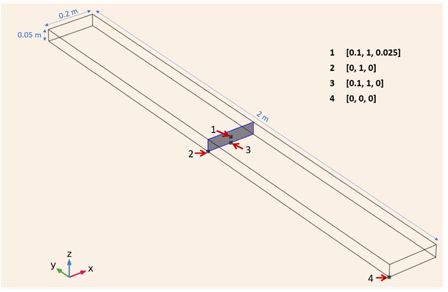Fig. 1. Geometry and dimensions of the timber; location of the special orthotropic body into the Cartesian coordinate system used in the model (= T, = L, = R), and position of points and area where the results were evaluated

The initial conditions were taken as uniform – constant in the whole volume. The initial moisture content value was lower than the fiber saturation point (M0 25%), which corresponds to the moisture content of pre‑dried timber. The uniform temperature of the timber at the drying process beginning was T0 283 K.

The properties of drying ambient and total time are given by the drying schedule. To be able to compare results of numerical simulations, the drying schedule has to be designed in accordance with the standard for wood kiln-drying (ON 49 0651 1989). The drying schedule parameters were set for the usual timber with final moisture M = 8%. The ambient parameters as RH and equilibrium moisture content (EMC) are given by the standard. Both are functions of time (Fig. 2). The air velocity and drying temperature are uniform throughout the drying process (vair 1.5 m/s, T = 353 K).

Each numerical model of the wood block was observed at four different points and at the middle area for values of temperature and moisture content during the drying time. The average moisture content in the whole body was observed.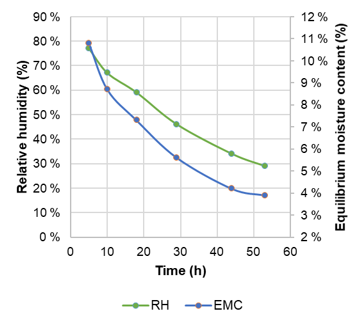Fig. 2. Drying schedule parameters during drying process

Coupled PDE’s Definition

The coupled transient modeling of the drying process is based on the system of two partial differential equations given by Siau (1992) and Avramidis et al. (1992b). The timber with an initial moisture content under the fiber saturation point contains the bound water. The motion of bound water is caused by diffusion. The main driving force of bound water diffusion is the moisture content gradient, but it is simultaneously influenced by the temperature gradient. Fick’s law describes the water diffusion problem. The first equation of the PDEs system (Eq. 1) is derived from Fick’s law and it is supplemented by a term of the Soret effect. The second PDE (2) is derived from Fourier’s law for transient thermal conduction. For the purposes of this study, the heat propagation is propelled only by the temperature gradient, the Duffour effect is neglected for its non-significant influence,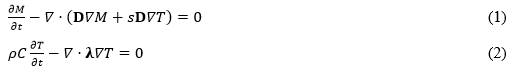where M is the actual moisture content (%), t is the time (s), D is the diffusion coefficients in the matrix (m2/s), s is the Soret effect coefficient (1/K), T is the actual temperature (K), ρ is the density (g/cm3), C is the specific heat (J/kg·K), and λ is the thermal conductivity coefficients matrix (W/m·K).

Applied boundary conditions, for moisture field (Eq. 3) and for thermal field (Eq. 4), are obtained from the heat and moisture transfer between the timber surfaces and the drying ambient (air). The boundary conditions are affected by the air velocity, which is considered in coefficients of heat and moisture transfer. These coefficients are invariable, induced in accordance with Siau (1995) and Horáček (2004) (pertaining to air velocity vair 1.5 m/s),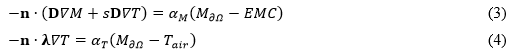where n is the normal vector, αM is the moisture transfer coefficient (m2/s), M is the boundary moisture content, EMC is the equilibrium moisture content, αT is the heat transfer coefficient (W/mK), T is the boundary temperature (K), and Tair is the air temperature (K).

Material Coefficients Definition

Material coefficients were defined as functions of moisture content and temperature. All of them were computed for every combination of moisture content values belonging to the interval M ∈ ⟨0; 0.3⟩ and temperature values from interval ∈ ⟨280; 380⟩. An analytical determination of all the material coefficients used in the most nonlinear numerical model B3 (Table 1) is presented below. The material coefficients and ambient parameters used in all four models are listed in Table 2.

Table 2. Ambient Parameters and Material Coefficients Used in Solved Numerical Models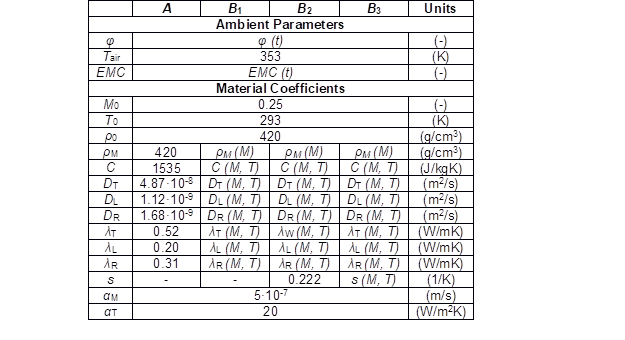Water diffusion coefficients

The water diffusion coefficients were computed separately for tangential (Eq. 5), longitudinal (Eq. 6), and radial (Eq. 7) directions. The computation of diffusion coefficients was based on formulas given by Siau (1995). Radial diffusion coefficient is 1.5× higher than the tangential diffusion coefficient (Trcala 2012),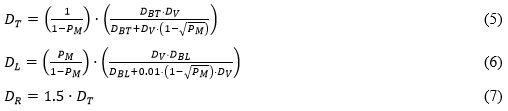where DT, DL, and DR are the diffusion coefficients for tangential, longitudinal, and radial direction (m2/s), respectively, PM is the wood porosity (-), DBT and DBL are the cell wall longitudinal and tangential diffusion coefficients (m2/s), respectively, and DV is the diffusion coefficient of water vapor in the cell lumen (m2/s).

Computations are based on Siau’s analytical model of water diffusion coefficients (Siau 1984), which was experimentally verified by Čermák and Trcala (2012). The formulas for diffusion coefficients computation are respecting two kinds of microstructural water motion– the diffusion in cell wall (Eqs. 8 and 9) and the diffusion in the cell lumen (Eq. 10). These formulas describe the longitudinal and transversal water diffusion in the cell wall. The value of longitudinal diffusion coefficient is higher than the value of the transversal one. This is caused by the divergent fibrillar structure of the cell wall in longitudinal and transversal directions (micro- and sub-microcapillaries oriented lengthwise with the cell wall axis) (Horáček 2004). Microstructural diffusion coefficients DBTDBL, and DV are nonlinear, affected by actual moisture content and temperature,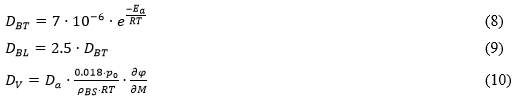where e is the Euler’s number (e = 2.71828), Ea is the activation energy of bound water (J/mol), R is the gas constant (= 8.3144 J/Kmol), Da is the air water vapor diffusion coefficient (m2/s), p0 is the saturated water vapor pressure (Pa), ρBS is the wood substance reduced density (g/cm3), and φ is the relative air humidity (-).

The activation energy of bound water in wood (Eq. 11) represents the influence of the actual moisture content on the cell wall diffusion coefficient (Skaar 1988).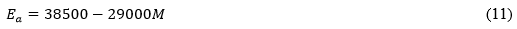The diffusion coefficient in cell lumen is based on the Dushman’s equation for water vapor diffusion in air (Eq. 12) (Dushman and Lafferty 1962). The dependence of cell lumen diffusion coefficient on temperature and moisture content is considered in formulas for saturated water vapor pressure (from Kirchhoff’s equation) (Siau 1995) (Eq. 13), reduced density of wood substance (Eq. 14), and RH (derived from DeBoer-Zwicker’s isotherm equation) (Eq. 15) (Siau 1995; Horáček 2004). The DeBoer-Zwicker’s coefficients for Picea sitchensis A (Eq. 16) and B (Eq. 17) were used to apply the temperature effect on RH computation (Horáček 2004). The used coefficient offers a sufficient approximation for wood of Picea abies (Babiak 1990),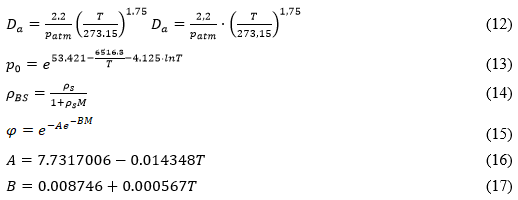where patm is the standard atmospheric pressure (patm = 101330 Pa), ρs is the wood substance density (ρs = 1500 g/cm3), and and are the DeBoer-Zwicker’s coefficients (-).

After the value of microstructural water diffusion coefficients, the next property that affects the diffusion coefficients is the wood porosity (Eq. 18). Wood porosity is dependent on the convective wood density (Eq. 19), which is the converted value of the dry wood density,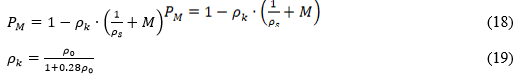where ρk is the conventional density (g/cm3) and ρ0 is the dry wood density (g/cm3).

The described theorems were applied for diffusion coefficients computation. The dependence of computed diffusion coefficients on temperature and moisture content is displayed in Fig. 3.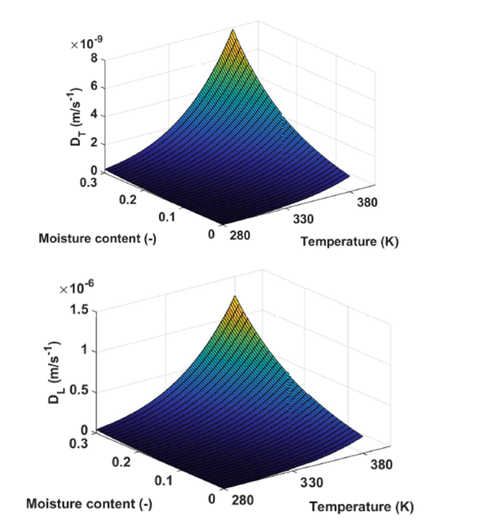Fig. 3. Tangential and longitudinal water-diffusion coefficients’ dependence on temperature and moisture content

Thermal conductivity coefficients

The thermal conductivity of wood is affected by its density, wood porosity, temperature, and moisture content. MacLean (1941) specified the equation (Eq. 20) for thermal conductivity of wood in the tangential direction. The thermal conductivity coefficients for radial (Eq. 21) and longitudinal (Eq. 22) directions gain 1.5× (R) and 2.5× (L) higher values than the tangential coefficient. In MacLean’s equation, there is a coefficient a, which is specified as a = 0.004 for M < 40%. The dependence of the thermal conductivity on the temperature was described as a linear function by Siau (1995) (Eq. 23).

Figure 4 presents the dependence of thermal conductivity in the tangential direction on temperature and moisture content,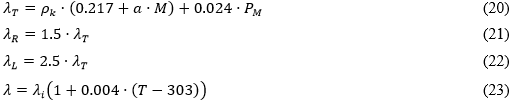where λTλR, and λT are the thermal conductivity coefficients (W/mK), and a is the coefficient for thermal conductivity determination (for < 0.4, a = 0.004).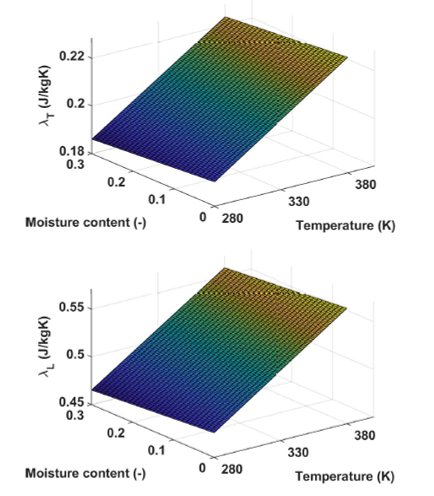Fig. 4. Tangential and longitudinal thermal conductivity coefficient’s dependence on temperature and moisture content

Specific heat

In the same way as previous material coefficients, the specific heat coefficient is a variable dependent on temperature and moisture content (Fig. 5). The formulas (Eqs. 24 and 25) defined by Skaar (1988) were used for specific heat calculations. Skaar’s definition of specific heat is valid for temperatures lower than 100 °C and for a moisture content lower than the fiber saturation point (ca 30%),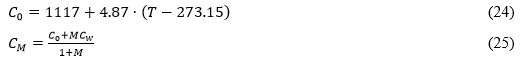where C0 is the specific heat of dry wood (J/kgK), CM is the specific heat of wood with actual moisture M (J/kgK), and Cis the specific heat of water (J/kgK).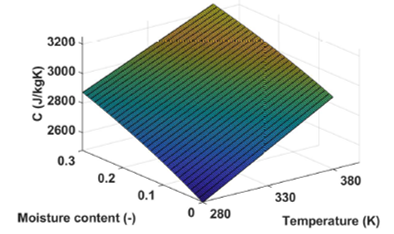Fig. 5. The dependence of specific heat coefficient on temperature and moisture content

Density

All of the simulations described in this paper were realized for wood of Picea abies. In the task of coupled moisture and heat transfer computations, the differences of the wood species is mainly expressed by its density. In this case, the density value of dried wood was ρ0 420 g/cm3. For numerical simulations, the density (Eq. 26) was defined as a function of moisture content. The following Kollmann’s equation (Kollmann 1951) is valid for moisture content lower than fiber saturation point and can be used with the assumption of the linear dependence of shrinkage on moisture content,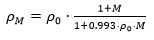(26)

where ρk is the density of wood with actual moisture (g/cm3).

Soret effect coefficient

The Soret effect coefficient (Eq. 27) reflects moisture flux incurred by the thermal gradient (Siau 1984; Avramidis et al. 1992b). It is based on the slope of the sorption isotherms and the activation energy for water-diffusion Ea (Eq. 11). The EMC is a function of relative humidity and the temperature (Eq. 28). The Soret effect decreases with the increase of moisture and with the increase of temperature (Fig. 6):